# KP-equation

(diff) ← Older revision | Latest revision (diff) | Newer revision → (diff)

The equation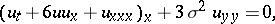with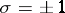. It arose in applied mathematics [a4] but was soon recognized to be related to problems of algebraic geometry and representation theory, besides spectral theory.

The general (formal) solution was found by M. Sato, cf. [a10], and is given as:by the tau-function, the section of a determinant line bundle over an infinite-dimensional Grassmannian; Sato proved that the KP-equation is equivalent to the Plücker relations for this Grassmannian.

The algebro-geometric solution was expressed by I.M. Krichever [a5] in terms of theta-functions on the Jacobi variety of a complex curve (cf. also Complex manifold) and T. Shiota [a11] settled the Novikov conjecture by proving that, conversely, if a theta-function satisfies the KP-equation, then its period matrix comes from a Riemann surface (cf. Schottky problem).

The spectral theory, an inverse scattering in two space and one time dimensions, was attacked most effectively by R. Beals and R.R. Coifman by designing the Neumann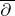-problem, cf. [a2].

All these methods treat also the KP-hierarchy, of which the KP-equation is the first non-trivial member. This hierarchy can be formulated for functions of infinitely many variablesas the identity for the coefficients of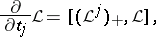whereis a (formal) pseudo-differential operator, the variables of the KP-equation are taken to be,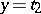,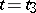,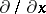is abbreviated asanddenotes deletion of the terms involving negative powers of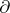. From this point of view, the curve associated to the algebro-geometric solutions is the spectral curve of the ring of differential operators inthat commute with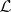and the times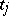are the isospectral deformations of the problem, which are linear flows on the Jacobi variety, a complex torus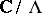; this theory was developed by J.L. Burchnall and T.W. Chaundy in the 1920s, cf. [a7].

The celebrated Korteweg–de Vries equation (respectively, the Boussinesq equation, see also Turbulence, mathematical problems in; Soliton; Oberbeck–Boussinesq equations) is but a reduction of the KP-equation, in the sense that the solution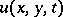is independent of(respectively,), as are other soliton equations ( cf. also Soliton), obtained by possibly modifying the group whose representation theory yields the tau-function (in the KP-case), cf. [a3].

Numerous issues related to the KP-equation are still under active investigation:

1) reality conditions (cf. [a9]), for the aforementioned solutions are given over the complex numbers;

2) solutions that belong to special classes of functions (cf. [a1]), such as rational, solitonic, elliptic, bispectral;

3) construction of solutions from vector bundles of rankover a curve (cf. [a6]), which generalize the Jacobian case where;

4) construction of analogous hierarchies for commuting matrix differential operators (cf. [a8]), whose spectral variety has dimension greater than; and

5) connections with matrix models and quantum field theory (cf. [a12]).

How to Cite This Entry:
KP-equation. Encyclopedia of Mathematics. URL: http://encyclopediaofmath.org/index.php?title=KP-equation&oldid=11256
This article was adapted from an original article by Emma Previato (originator), which appeared in Encyclopedia of Mathematics - ISBN 1402006098. See original article